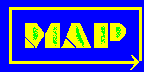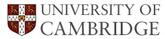# Materials Algorithms ProjectCrystallography: Subroutines LibraryThis index contains SUBBROUTINES relating to the modelling of crystallographic phenomena.

Format of documentation within this library.

[A][B][C][D] [E][F][G][H] [IJ][K][L][M] [N][O][PQ][R] [S][T][UVW][XYZ]

### A

MAP_CRYSTAL_ANGLEE
Finds the angle between two vectors.
Language: FORTRAN

[Top]

[Top]

### C

MAP_CRYSTAL_CONVERT
Converts the components of a vector from real to reciprocal space, or vice versa.
Language: FORTRAN

MAP_CRYSTAL_CORD
Calculates the coordinate transformation matrix relating two crystals of arbitrary structure.
Language: FORTRAN

[Top]

### D

MAP_CRYSTAL_DSP
Obtains the spacing of planes, when the Miller indices are not those which are systematically absent.
Language: FORTRAN

MAP_CRYSTAL_DSPACE
Obtains the spacing of planes.
Language: FORTRAN

[Top]

### E

MAP_CRYSTAL_ED1
Performs electron diffraction analysis in cases where the camera constant is known.
Language: FORTRAN

MAP_CRYSTAL_ED2
Performs electron diffraction analysis in cases where the camera constant is unknown.
Language: FORTRAN

[Top]

[Top]

[Top]

[Top]

[Top]

[Top]

[Top]

### M

MAP_CRYSTAL_MET1
Calculates the metric tensor for a cubic crystal structure.
Language: FORTRAN

MAP_CRYSTAL_MET2
Calculates the metric tensor for a tetragonal crystal structure.
Language: FORTRAN

MAP_CRYSTAL_MET3
Calculates the metric tensor for an orthorhombic crystal structure.
Language: FORTRAN

MAP_CRYSTAL_MET4
Calculates the metric tensor for a hexagonal or trigonal crystal structure.
Language: FORTRAN

MAP_CRYSTAL_MET5
Calculates the metric tensor for a monoclinic crystal structure.
Language: FORTRAN

MAP_CRYSTAL_MET6
Calculates the metric tensor for a triclinic crystal.
Language: FORTRAN

[Top]

### N

MAP_CRYSTAL_NOTAT1
Converts from four index notation to three index notation for a hexagonal lattice.
Language: FORTRAN

MAP_CRYSTAL_NOTAT2
Converts from three index notation to four index notation for a hexagonal lattice.
Language: FORTRAN

[Top]

### O

MAP_CRYSTAL_ORIENT
Contains rotation matrices defining the symmetry operations of a cubic lattice.
Language: FORTRAN

[Top]

### PQ

MAP_CRYSTAL_PAIR
Calculates all 24 symmetry-related axis-angle pairs relating two cubic lattices from an input consisting of a pair of vectors from each crystal and an angle between the two sets.
Language: FORTRAN

[Top]

### R

MAP_CRYSTAL_ROTAT
Calculates the 23 axis-angle pairs which are equivalent to a given axis-angle pair in a cubic lattice.
Language: FORTRAN

[Top]

[Top]

### T

MAP_CRYSTAL_TENSOR1
Calculates the metric tensor and its inverse for a cubic crystal.
Language: FORTRAN

MAP_CRYSTAL_TENSOR2
Calculates the metric tensor and its inverse for a tetragonal crystal.
Language: FORTRAN

MAP_CRYSTAL_TENSOR3
Calculates the metric tensor and its inverse for an orthorhombic crystal.
Language: FORTRAN

MAP_CRYSTAL_TENSOR4
Calculates the metric tensor and its inverse for a hexagonal or trigonal crystal.
Language: FORTRAN

MAP_CRYSTAL_TENSOR5
Calculates the metric tensor and its inverse for a monoclinic crystal.
Language: FORTRAN

MAP_CRYSTAL_TENSOR6
Calculates the metric tensor and its inverse for a triclinic crystal.
Language: FORTRAN

MAP_CRYSTAL_TEST1
Calculates the angle between two reciprocal lattice vectors, and the magnitude of those vectors.
Language: FORTRAN

MAP_CRYSTAL_TEST2
As MAP_CRYSTAL_TEST1.
Language: FORTRAN

MAP_CRYSTAL_TYPE
Checks for systematic absences in various lattice types.
Language: FORTRAN

[Top]

### UVW

MAP_CRYSTAL_VECMAG
Calculates the magnitude of a vector defined in real space.
Language: FORTRAN

MAP_CRYSTAL_VOL
Calculates the unit cell volume from the metric tensor.
Language: FORTRAN

[Top]

[Top]